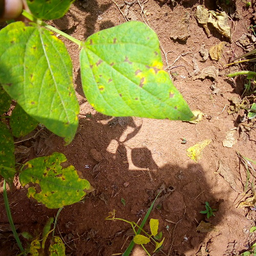# 基于 HuggingFace Datasets 和 Transformers 的图像相似性搜索

## 计算嵌入

``````from transformers import AutoFeatureExtractor, AutoModel

model_ckpt = "nateraw/vit-base-beans"
extractor = AutoFeatureExtractor.from_pretrained (model_ckpt)
model = AutoModel.from_pretrained (model_ckpt)
``````

Q1: 为什么我们不使用 `AutoModelForImageClassification`

Q2: 为什么使用这个特定的 checkpoint？

## 加载候选图像数据集

``````from datasets import load_dataset

````````````dataset ["train"].features
>>> {'image_file_path': Value (dtype='string', id=None),
'image': Image (decode=True, id=None),
'labels': ClassLabel (names=['angular_leaf_spot', 'bean_rust', 'healthy'], id=None)}
``````

``````num_samples = 100
seed = 42
candidate_subset = dataset ["train"].shuffle (seed=seed).select (range (num_samples))
``````

## 寻找相似图片的过程

1. 从候选图像 (`candidate_subset`) 中提取嵌入，将它们存储在一个矩阵中。
2. 获取查询图像并提取其嵌入。
3. 遍历嵌入矩阵 (步骤 1 中得到的) 并计算查询嵌入和当前候选嵌入之间的相似度得分。我们通常维护一个类似字典的映射，来维护候选图像的 ID 与相似性分数之间的对应关系。
4. 根据相似度得分进行排序并返回相应的图像 ID。最后，使用这些 ID 来获取候选图像。

``````import torch

def extract_embeddings (model: torch.nn.Module):
"""Utility to compute embeddings."""
device = model.device

def pp (batch):
images = batch ["image"]
# `transformation_chain` is a compostion of preprocessing
# transformations we apply to the input images to prepare them
# for the model. For more details, check out the accompanying Colab Notebook.
image_batch_transformed = torch.stack (
[transformation_chain (image) for image in images]
)
new_batch = {"pixel_values": image_batch_transformed.to (device)}
embeddings = model (**new_batch).last_hidden_state [:, 0].cpu ()
return {"embeddings": embeddings}

return pp
``````

``````device = "cuda" if torch.cuda.is_available () else "cpu"
extract_fn = extract_embeddings (model.to (device))
candidate_subset_emb = candidate_subset.map (extract_fn, batched=True, batch_size=batch_size)
``````

``````candidate_ids = []

for id in tqdm (range (len (candidate_subset_emb))):
label = candidate_subset_emb [id]["labels"]

# Create a unique indentifier.
entry = str (id) + "_" + str (label)

candidate_ids.append (entry)
``````

``````all_candidate_embeddings = np.array (candidate_subset_emb ["embeddings"])
all_candidate_embeddings = torch.from_numpy (all_candidate_embeddings)
``````

``````def compute_scores (emb_one, emb_two):
"""Computes cosine similarity between two vectors."""
scores = torch.nn.functional.cosine_similarity (emb_one, emb_two)
return scores.numpy ().tolist ()

def fetch_similar (image, top_k=5):
"""Fetches the`top_k`similar images with`image`as the query."""
# Prepare the input query image for embedding computation.
image_transformed = transformation_chain (image).unsqueeze (0)
new_batch = {"pixel_values": image_transformed.to (device)}

# Comute the embedding.
query_embeddings = model (**new_batch).last_hidden_state [:, 0].cpu ()

# Compute similarity scores with all the candidate images at one go.
# We also create a mapping between the candidate image identifiers
# and their similarity scores with the query image.
sim_scores = compute_scores (all_candidate_embeddings, query_embeddings)
similarity_mapping = dict (zip (candidate_ids, sim_scores))

# Sort the mapping dictionary and return `top_k` candidates.
similarity_mapping_sorted = dict (
sorted (similarity_mapping.items (), key=lambda x: x , reverse=True)
)
id_entries = list (similarity_mapping_sorted.keys ())[:top_k]

ids = list (map (lambda x: int (x.split ("_")), id_entries))
labels = list (map (lambda x: int (x.split ("_")[-1]), id_entries))
return ids, labels
``````

## 执行查询

``````test_idx = np.random.choice (len (dataset ["test"]))
test_sample = dataset ["test"][test_idx]["image"]
test_label = dataset ["test"][test_idx]["labels"]

sim_ids, sim_labels = fetch_similar (test_sample)
print (f"Query label: {test_label}")
print (f"Top 5 candidate labels: {sim_labels}")
``````

``````Query label: 0
Top 5 candidate labels: [0, 0, 0, 0, 0]
``````

## 进一步扩展与结论

• 如果我们按原样存储嵌入，内存需求会迅速增加，尤其是在处理数百万张候选图像时。在我们的例子中嵌入是 768 维，这即使对大规模系统而言可能也是相对比较高的维度。
• 高维的嵌入对检索部分涉及的后续计算有直接影响。Datasets 提供与 FAISS 的直接集成，进一步简化了构建相似性系统的过程。假设你已经提取了候选图像的嵌入 (`beans` 数据集) 并把他们存储在称为 `embedding``feature` 中。你现在可以轻松地使用 `dataset``add_faiss_index()` 方法来构建稠密索引:

``````dataset_with_embeddings.add_faiss_index (column="embeddings")
``````

``````scores, retrieved_examples = dataset_with_embeddings.get_nearest_examples (
"embeddings", qi_embedding, k=top_k
)
``````